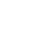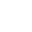> > Introduction to Conic Sections

# Introduction to Conic Sections

Conic Sections: The term “conic” is derived from the word “cone” and as the name suggests, we are going to cut the cone out in different sections. Each type of section will have its own defining properties. A cone is an interesting shape which is very familiar in our day-to-day lives, like an ice-cream cone, the birthday hat etc. Let’s explore the cones and find out what exciting things are hidden inside a conic section.

#### VideosIntroduction to Conic SectionsTerms Associated to ConicsDiameter of a Parabola## Elements of a Conic

The conic sections can be formed by the intersection of a right circular cone and a plane in different ways. For a better idea, take a look at the image belowStraight lines                            Circle                          Parabola.                Ellipse                    Hyperbola

## Terminology in Conic Section

• Generator: It’s a straight line running from the apex to the base of a cone.
• Axis: It’s a straight line which runs from the apex of the cone to the centre of the base.
• Conic: It’s the locus of a point which moves in a plane, such that: the ratio of its distance from a fixed point to its perpendicular distance from a fixed straight line is always constant.
• Focus: The fixed point is the focus of the conic.
• Directrix: The fixed straight line is the directrix of the conic.
• Eccentricity: The constant ratio is the eccentricity of the conic, denoted by e.
• Vertex: The intersection points of the conic and the axis is (are) called vertex (vertices).
• Focal chord: Any chord passing through the focus is called the focal chord
• Centre: The point which bisects every chord of the conic passing through it, is called the centre.
• Double ordinate: It’s a straight line which is perpendicular to the axis and terminated at both ends of the curve.
• Latus rectum: It’s the double ordinate which passes through the focus.## Eccentricity

The eccentricity of a conic determines how uncircular a curve is. Like for a circle, the eccentricity is 0 because it is circular. Similarly, an ellipse is the closest look-alike of a circle and hence its value is greater than 0 but less than 1. Eccentricity is basically a ratio of the distance of focus from a fixed point to its perpendicular distance from a fixed straight line, which is constant.

In the table below you’ll find the values of eccentricity for different conics –

 Eccentricity Conic $$e=1$$ Parabola $$e<1$$ Ellipse $$e>1$$ Hyperbola $$e=0$$ Circle $$e=∞$$ Pair of Straight Lines

## General Equation of a Conic Section

The general equation that represents any conic is given as –

$$ax^2+2hxy+by^2+2gx+2fy+c=0$$

Here, the following conditions govern which conic will be represented from the above equation based on Δ where

$$Δ=abc+2fgh−af^2−bg^2−ch^2$$

Let us take a look at the following cases –

Case 1: When Δ=0, the equation represents the degenerate conics.

 Condition Conic $$ab−h^2=0$$ Straight line $$ab−h^2≠0$$ Pair of intersecting straight lines $$ab−h^2<0$$ Real or imaginary pair of straight lines $$ab−h^2>0$$ Point

Case 2: When Δ≠0, the equation represents the non−degenerate conics.

 Condition Conic $$h=0,a=b$$ Circle $$ab−h^2=0$$ Parabola $$ab−h^2>0$$ Ellipse $$ab−h^2<0$$ Hyperbola $$ab−h^2<0,a+b=0$$ Rectangular Hyperbola

*A degenerate conic is a conic which cannot be reduced into a curve. It means that the defining equation will get factored into the complex numbers as the product of two linear polynomials.

## Centre of a Conic Section

The centre of a conic represented by the equation $$ax^2+2hxy+by^2+2gx+2fy+c=0$$ is given by

## Solved Examples for Conic Sections

Question 1: For k=0, the equation $$2x^2+3y^2−8x−18y+35=k$$ represents?

Answer : $$2x^2+3y^2−8x−18y+35=k$$

This can be written as $$2(x−2)^2+3(y−3)^2=k$$

Thus, if k=0, the equation will represent a point.

If k>0, it will be an ellipse.

If k<0, then there will be no locus.

Question 2: What are conic sections?

Answer:A circle, parabolas, ellipses, and hyperbolas are known as conic sections because intersecting a right circular cone with a plane can form them. In addition, when the plane is perpendicular to the axis of the cone, the resulting intersection is a circle.

Question 3: What are the four conic sections?

Answer: A conic section refers to an intersection of a plane and a double right circular cone. Moreover, by changing the angle and location of the intersection, we can produce different types of conics. How ever, the basic four types are ellipses, hyperbolas, parabolas, and circle.

Question 4: Why are conic sections important?

Answer: They are important because with their help we can study 3D (three-dimensional) geometry that has wide application. In addition, it helps in electromagnetic field theory to study the nature of the field inside different shapes of conductors.

Question 5: Is half of the ellipses is a parabola?

Answer: When you slice it with a slightly tilted plane, you’ll get an ellipse (or a single point). Therefore, circles and ellipses are both ‘cross-sections’ of a cone or “conic sections.” Most importantly, at that tilt, the intersection is no longer an ellipse instead it is a parabola. Hence, we can say that parabola is the limit of ellipses.

Share with friends

## Customize your course in 30 seconds

##### Which class are you in?
5th
6th
7th
8th
9th
10th
11th
12th
Get ready for all-new Live Classes!
Now learn Live with India's best teachers. Join courses with the best schedule and enjoy fun and interactive classes.Ashhar Firdausi
IIT Roorkee
BiologyDr. Nazma Shaik
VTU
ChemistryGaurav Tiwari
APJAKTU
Physics
Get Started

## Browse

##### Conic Sections2 Followers

Most reacted comment
1 Comment authorsRecent comment authors
Subscribe
Notify ofGuest
Dami

Nigeria not among

## Question Mark?

Have a doubt at 3 am? Our experts are available 24x7. Connect with a tutor instantly and get your concepts cleared in less than 3 steps.